### An Introduction to the Kalman-Bucy Filter

The Kalman-Bucy Filter is a continuous time counterpart to the discrete time Kalman Filter. As with the Kalman Filter, the Kalman-Bucy Filter is designed to estimate unmeasured states of a process, usually for the purpose of controlling one or more of them.

Other tutorials discuss the Kalman Filter and its non-linear forms -- the Extended Kalman Filter and the Unscented Kalman Filter.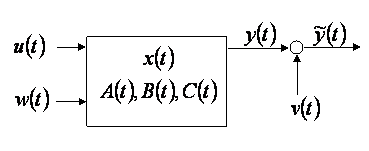Figure 1: Linear continuous-time process with input and measurement noise.

Consider the linear continuous-time process shown in Figure 1 which may be written in the following standard state-space form. (The time dependence of the parameters has been dropped to simplify the notation.)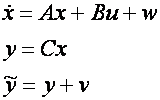Here

• u is the vector of inputs
• x is a vector of the actual states, which may be observable but not measured.
• y is a vector of the actual process outputs.
•is a vector of the measured process outputs.
• w and v are process and output noise respectively. They are assumed to be zero mean Gaussian with covariance Q and R respectively.

Given the inputs, measured outputs and assumptions on the process and output noise, the purpose of the Kalman-Bucy Filter is to estimate unmeasured states (assuming they are observable) and the actual process outputs. This is shown in Figure 2 where the estimated states are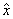, andare the estimated measured outputs.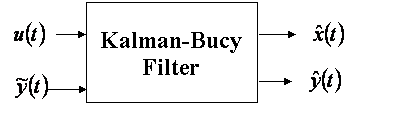Figure 2: Input-output of the Kalman-Bucy Filter.

Unlike the Kalman Filter the Kalman-Bucy filter does not use a predictor-corrector algorithm to update the state estimates. Rather it requires a differential Riccati equation to be integrated through time.

Mathematically, the filter update equations are given by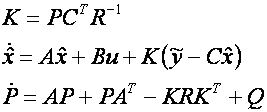In the above equations P is an estimate of the covariance of the measurement error and K is called the Kalman-Bucy gain. As part of the filter implementation bothand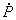must be integrated through time. Note that since P is a symmetric matrix the number of covariance states that must be integrated may be reduced by only considering the diagonal and terms above (or below) the diagonal.

A simple example demonstrating how to implement a Kalman-Bucy filter in Simulink can be found here.